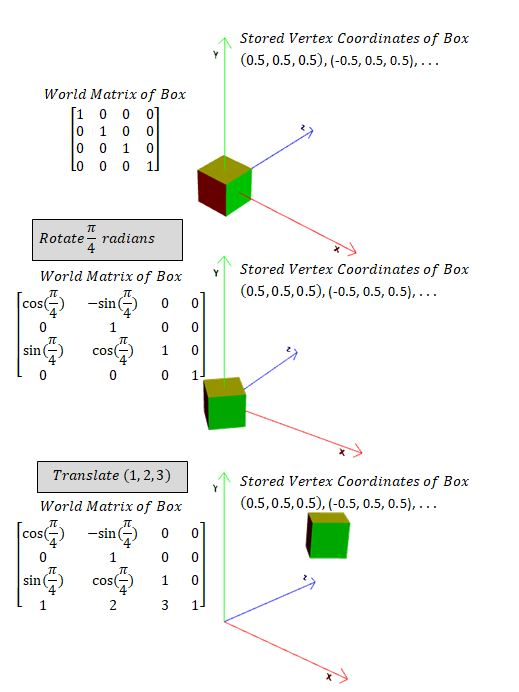# Frame of Reference

Every mathematical vector and transformation is expressed in a frame of reference which is stored as a matrix. In Babylon.js the data describing a mesh is stored as local space vectors. The frame of reference for the mesh is determined by the world matrix for the mesh which is formed from any rotation, translation and scaling operations. For each rendered frame the current world matrix is used on the local space data to obtain the world data for the mesh.

The vertex data for a mesh on creation is stored and remains the same throught Babylon.js processing unless baked. As you position, translate, rotate and scale a mesh the world matrix for the mesh is updated to reflect these transformations. Before rendering each frame the world matrix is applied to the mesh vertex data to determine its world 3D data. However the frame of reference for the mesh to be viewed is a 2D screen and this frame of reference is a projection matrix which is applied to the mesh's world 3D data.

The world and projection matrix operations are carried out within the GPU (graphic processor unit) in a piece of code called the vertex shader.Though rarely needed you can set the vertex data of the mesh to its world 3D data and either reset the world matrix to the identity matrix or leave it be. This is done by baking the transformation into the mesh.

# Coordinate Transformation Explained

The TransformCoordinates function takes a position vector in one frame of reference and places it in another frame of reference using the matrix that transforms one frame of reference into the other.

You are able to use the world matrix of a mesh to transform position vectors, and only position vectors, from a mesh's local axes coordinates to the world axes coordinates. For example

``````var matrix = mesh.computeWorldMatrix(true); //true forces a recalculation rather than using cache version
var local_position = new BABYLON.Vector3(0,1,0);
var global_position = BABYLON.Vector3.TransformCoordinates(local_position, matrix);
``````

Should you want to translate the local_position, in the above example, its current local position of (0, 1, 0) by (1, 1, 1) then this must be done to the local position before applying `TransformCoodinates` since this only transforms position vectors not direction vectors.

``````var matrix = mesh.computeWorldMatrix(true);
var local_position = new BABYLON.Vector3(0,1,0);
Potential uses of `BABYLON.Vector3.TransformCoordinates()` may be: